Next: An example 2-d Poisson Up: Poisson's equation Previous: 2-d problem with Neumann

## The fast Fourier transform

The method outlined in Sect. 5.7 for solving Poisson's equation in 2-d with simple Dirichlet boundary conditions in the-direction requires us to perform very many Fourier-sine transforms: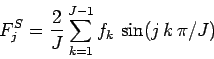(169)

for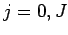, and inverse Fourier-sine transforms: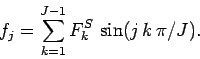(170)

Here,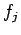is the value of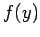at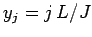. Thus, Eq. (169) is analogous to Eqs. (153) and (156), whereas Eq. (170) can be used to reconstruct the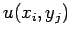from the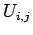. Likewise, the method outlined in Sect. 5.8 for solving Poisson's equation in 2-d with simple Neumann boundary conditions in the-direction requires us to perform very many Fourier-cosine transforms: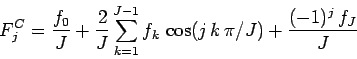(171)

for, and inverse Fourier-cosine transforms: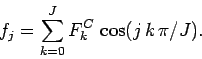(172)

Unfortunately, performing such transforms directly requires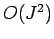arithmetic operations, which means that they are extremely expensive in terms of cpu resources. There is, however, an ingenious algorithm for performing Fourier transforms which only takes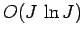arithmetic operations [which is much less thanoperations when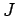is large]. This algorithm is known as the fast Fourier transform or FFT.35

The details of the FFT algorithm lie beyond the scope of this course. Roughly speaking, the algorithm works by building up the transform in stages using 2, 4, 8, 16, etc. grid-points. In this course, we shall employ the best-known publicly available FFT library, called the fftw library,36 to perform all of our Fourier-sine and -cosine transforms. Unfortunately, the fftw library does not directly calculate Fourier-sine and -cosine transforms.37 Instead, it calculates complex Fourier transforms: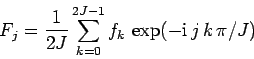(173)

for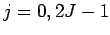, and complex inverse Fourier transforms: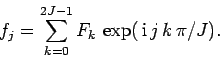(174)

Note thatand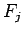are periodic in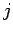with period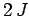. Note, further, that the data-sets associated with complex Fourier transforms contain twice as many elements as the data-sets associated with sine and cosine transforms. However, we can easily convert a sine or cosine transform into a complex transform by extending its data-set. Thus, for a sine transform we write: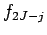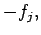(175)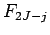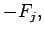(176)

for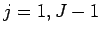, in which case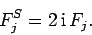(177)

Likewise, for a cosine transform we write: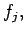(178)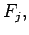(179)

for, in which case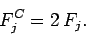(180)

Listed below are a set of wrapper routines which employ the fftw library to perform Fourier-sine and -cosine transforms.

// FFT.cpp

// Set of functions to calculate Fourier-cosine and -sine transforms
// of real data using fftw Fast-Fourier-Transform library.
// Input/ouput arrays are assumed to be of extent J+1.
// Uses version 2 of fftw library (incompatible with vs 3).

#include <fftw.h>
#include <blitz/array.h>

using namespace blitz;

// Calculates Fourier-cosine transform of array f in array F
void fft_forward_cos (Array<double,1> f, Array<double,1>& F)
{
// Find J. Declare local arrays.
int J = f.extent(0) - 1;
int N = 2 * J;
fftw_complex ff[N], FF[N];

// Load and extend data
c_re (ff) = f(0); c_im (ff) = 0.;
c_re (ff[J]) = f(J); c_im (ff[J]) = 0.;
for (int j = 1; j < J; j++)
{
c_re (ff[j]) = f(j); c_im (ff[j]) = 0.;
c_re (ff[2*J-j]) = f(j); c_im (ff[2*J-j]) = 0.;
}

// Call fftw routine
fftw_plan p = fftw_create_plan (N, FFTW_FORWARD, FFTW_ESTIMATE);
fftw_one (p, ff, FF);
fftw_destroy_plan (p);

// Unload data
F(0) = c_re (FF); F(J) = c_re (FF[J]);
for (int j = 1; j < J; j++)
{
F(j) = 2. * c_re (FF[j]);
}

// Normalize data
F /= 2. * double (J);
}

// Calculates inverse Fourier-cosine transform of array F in array f
void fft_backward_cos (Array<double,1> F, Array<double,1>& f)
{
// Find J. Declare local arrays.
int J = f.extent(0) - 1;
int N = 2 * J;
fftw_complex ff[N], FF[N];

// Load and extend data
c_re (FF) = F(0); c_im (FF) = 0.;
c_re (FF[J]) = F(J); c_im (FF[J]) = 0.;
for (int j = 1; j < J; j++)
{
c_re (FF[j]) = F(j) / 2.; c_im (FF[j]) = 0.;
FF[2*J-j] = FF[j];
}

// Call fftw routine
fftw_plan p = fftw_create_plan (N, FFTW_BACKWARD, FFTW_ESTIMATE);
fftw_one (p, FF, ff);
fftw_destroy_plan (p);

// Unload data
f(0) = c_re (ff); f(J) = c_re (ff[J]);
for (int j = 1; j < J; j++)
{
f(j) = c_re (ff[j]);
}
}

// Calculates Fourier-sine transform of array f in array F
void fft_forward_sin (Array<double,1> f, Array<double,1>& F)
{
// Find J. Declare local arrays.
int J = f.extent(0) - 1;
int N = 2 * J;
fftw_complex ff[N], FF[N];

// Load and extend data
c_re (ff) = 0.; c_im (ff) = 0.;
c_re (ff[J]) = 0.; c_im (ff[J]) = 0.;
for (int j = 1; j < J; j++)
{
c_re (ff[j]) = f(j); c_im (ff[j]) = 0.;
c_re (ff[2*J-j]) = - f(j); c_im (ff[2*J-j]) = 0.;
}

// Call fftw routine
fftw_plan p = fftw_create_plan (N, FFTW_FORWARD, FFTW_ESTIMATE);
fftw_one (p, ff, FF);
fftw_destroy_plan (p);

// Unload data
F(0) = 0.; F(J) = 0.;
for (int j = 1; j < J; j++)
{
F(j) = - 2. * c_im (FF[j]);
}

// Normalize data
F /= 2. * double (J);
}

// Calculates inverse Fourier-sine transform of array F in array f
void fft_backward_sin (Array<double,1> F, Array<double,1>& f)
{
// Find J. Declare local arrays.
int J = f.extent(0) - 1;
int N = 2 * J;
fftw_complex ff[N], FF[N];

// Load and extend data
c_re (FF) = 0.; c_im (FF) = 0.;
c_re (FF[J]) = 0.; c_im (FF[J]) = 0.;
for (int j = 1; j < J; j++)
{
c_re (FF[j]) = 0.; c_im (FF[j]) = - F(j) / 2.;
c_re (FF[2*J-j]) = 0.; c_im (FF[2*J-j]) = F(j) / 2.;
}

// Call fftw routine
fftw_plan p = fftw_create_plan (N, FFTW_BACKWARD, FFTW_ESTIMATE);
fftw_one (p, FF, ff);
fftw_destroy_plan (p);

// Unload data
f(0) = 0.; f(J) = 0.;
for (int j = 1; j < J; j++)
{
f(j) = c_re (ff[j]);
}
}Next: An example 2-d Poisson Up: Poisson's equation Previous: 2-d problem with Neumann
Richard Fitzpatrick 2006-03-29